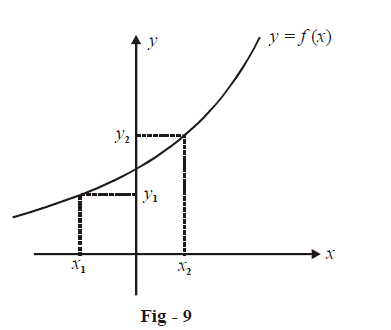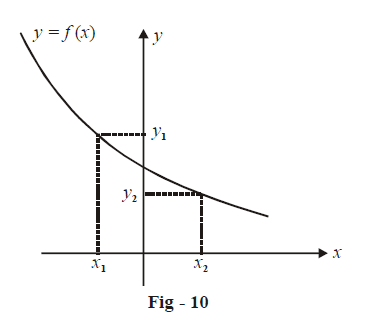# Introduction To Monotonicity

Go back to  'Applications of Derivatives'

In this section, we turn our attention to the increasing / decreasing nature of functions and how the concept of derivatives can help us in determining this nature.

Consider a function represented by the following graph:For two different input arguments $${x_1}\;and\;{x_2},$$ where $${x_1} < {x_2}, \, {y_1} = f\left( {{x_1}} \right)$$ will always be less than $${y_2} = f\left( {{x_2}} \right)$$ .

That is,

${x_1} < {x_2}{\rm{ \;\;\; implies \;\;\; }}f\left( {{x_1}} \right) < f\left( {{x_2}} \right)$

Such a function is called a strictly increasing function or a monotonically increasing function (The word ‘monotonically’ apparently has its origin in the word monotonous; for example, a monotonous routine is one in which one follows the same routine repeatedly or continuously; similarly a monotonically increasing function is one that increases continuously).

Now, consider \begin{align}f\left( x \right) = \left[ x \right].\end{align} For this function

$${x_1} < {x_2}\;\text{does not always imply}f\left( {{x_1}} \right) < f\left( {{x_2}} \right)$$

However,              $${x_1} < {x_2}$$  does imply $$f\left( {{x_1}} \right) \le f\left( {{x_2}} \right)$$

In other words, $$f\left( x \right) = \left[ x \right]$$ is not strictly (or monotonically) increasing. It will nevertheless be termed increasing.

Now consider a function represented by the following graph:For two different input arguments $${x_1}\;and\;{x_2},$$ where $${x_1} < {x_2},{y_1} = f\left( {{x_1}} \right)$$ will always be greater than  $${y_2} = f\left( {{x_2}} \right)$$ .

That is,

${x_1} < {x_2} \Rightarrow f\left( {{x_1}} \right) > f\left( {{x_2}} \right)$

Such a function is called a strictly decreasing function or a monotonically decreasing function.

\begin{align}\;\;\text{Now consider}\;\;&f\left( x \right) = - \left[ x \right] . \text{For this function}\\\\&{x_1} < {x_2}\;\text{does not imply} \;f\left( {{x_1}} \right) > f\left( {{x_2}} \right)\\\\However, \quad &{x_1} < {x_2} \qquad \qquad \Rightarrow \qquad f\left( {{x_1}} \right) \ge f\left( {{x_2}} \right)\end{align}

Therefore, $$f\left( x \right) = - \left[ x \right]$$ is not strictly decreasing. It would only be termed decreasing.

The following table lists down a few examples of functions and their behaviour in different intervals. You are urged to verify all the assertions listed on your own.

 Function Behaviour $$f\left( x \right) = x$$ : Strictly increasing on $$\mathbb{R}$$ $$f\left( x \right) = {x^2}$$ : Strictly decreasing on $$\left( { - \infty ,0} \right]$$ Strictly increasing on $$\left[ {0,\infty } \right)$$ $$f\left( x \right) = \sqrt x$$ : Strictly increasing on $$\left[ {0,\infty } \right)$$ $$f\left( x \right) = {x^3}$$ : Strictly increasing on $$\mathbb{R}$$ $$f\left( x \right) = \left| x \right|$$ : Strictly decreasing on $$\left( { - \infty ,0} \right]$$ Strictly increasing on $$\left[ {0,\infty } \right)$$ \begin{align}f\left( x \right) = \frac{1}{x}\end{align} : Neither increasing nor decreasing on $$\mathbb{R} .$$ Strictly decreasing on $$\left( { - \infty ,0} \right)$$ Strictly decreasing on $$\left( {0,\infty } \right)$$ $$f\left( x \right) = \left[ x \right]$$ : Increasing on $$\mathbb{R}$$ $$f\left( x \right) = \left\{ x \right\}$$ : Neither increasing nor decreasing on $$\mathbb{R}.$$ However, strictly increasing on $$\left[ {n,n + 1} \right)$$ where $$n \in \mathbb{Z}$$ $$f\left( x \right) = \sin x$$ : Neither increasing nor decreasing on$$\mathbb{R}.$$ Strictly increasing on \begin{align}[(\,2n - \frac{1}{2}\,)\pi ,(2n + \frac{1}{2})\pi ];n \in \mathbb{Z}\end{align} Strictly decreasing on \begin{align}[(2n + \frac{1}{2})\pi ,(2n + \frac{3}{2})\pi ];n \in \mathbb{Z}\end{align} $$f\left( x \right) = \cos x$$ : Neither increasing nor decreasing on $$\mathbb{R}.$$ Strictly increasing on $$[(2n - 1)\pi ,2n\pi ];n \in \mathbb{Z}$$ Strictly decreasing on $$[2n\pi ,(2n + 1)\pi ];n \in \mathbb{Z}$$ $$f\left( x \right) = \tan x$$ : Neither increasing nor decreasing on $$\mathbb{R}.$$ Strictly increasing on \begin{align}\left( {\left( {n - \frac{1}{2}} \right)\pi ,\left( {n + \frac{1}{2}} \right)\pi } \right);n \in \mathbb{Z}\end{align} $$f\left( x \right) = {e^x}$$ : Strictly increasing on $$\mathbb{R}$$ $$f\left( x \right) = {e^{ - x}}$$ : Strictly decreasing on $$\mathbb{R}$$ $$f\left( x \right) = \ln x$$ : Strictly increasing on $$\left( {0,\infty } \right)$$